Search by Topic

Resources tagged with Multiplication & division similar to The Brown Family:

Filter by: Content type:
Age range:
Challenge level:

There are 116 results

Broad Topics > Calculations and Numerical Methods > Multiplication & division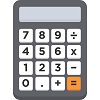Secret Number

Age 5 to 7 Challenge Level:

Annie and Ben are playing a game with a calculator. What was Annie's secret number?The Brown Family

Age 5 to 7 Challenge Level:

Use the information about Sally and her brother to find out how many children there are in the Brown family.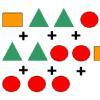Super Shapes

Age 7 to 11 Short Challenge Level:

The value of the circle changes in each of the following problems. Can you discover its value in each problem?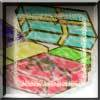Age 7 to 11 Challenge Level:

Use the information to work out how many gifts there are in each pile.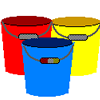Buckets of Thinking

Age 7 to 11 Challenge Level:

There are three buckets each of which holds a maximum of 5 litres. Use the clues to work out how much liquid there is in each bucket.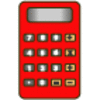Clever Keys

Age 7 to 11 Short Challenge Level:

On a calculator, make 15 by using only the 2 key and any of the four operations keys. How many ways can you find to do it?One Million to Seven

Age 7 to 11 Challenge Level:

Start by putting one million (1 000 000) into the display of your calculator. Can you reduce this to 7 using just the 7 key and add, subtract, multiply, divide and equals as many times as you like?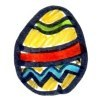How Many Eggs?

Age 7 to 11 Challenge Level:

Peter, Melanie, Amil and Jack received a total of 38 chocolate eggs. Use the information to work out how many eggs each person had.Sam's Quick Sum

Age 7 to 11 Challenge Level:

What is the sum of all the three digit whole numbers?The Clockmaker's Birthday Cake

Age 7 to 11 Challenge Level:

The clockmaker's wife cut up his birthday cake to look like a clock face. Can you work out who received each piece?Looking at Lego

Age 7 to 11 Challenge Level:

This task offers an opportunity to explore all sorts of number relationships, but particularly multiplication.Birthday Sharing

Age 5 to 7 Challenge Level:

It's Sahila's birthday and she is having a party. How could you answer these questions using a picture, with things, with numbers or symbols?A Conversation Piece

Age 7 to 11 Challenge Level:

Take the number 6 469 693 230 and divide it by the first ten prime numbers and you'll find the most beautiful, most magic of all numbers. What is it?Catrina's Cards

Age 5 to 7 Challenge Level:

How would you find out how many football cards Catrina has collected?Difficulties with Division

Age 5 to 11

This article for teachers looks at how teachers can use problems from the NRICH site to help them teach division.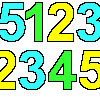1, 2, 3, 4, 5

Age 7 to 11 Challenge Level:

Using the numbers 1, 2, 3, 4 and 5 once and only once, and the operations x and ÷ once and only once, what is the smallest whole number you can make?Learning Times Tables

Age 5 to 11 Challenge Level:

In November, Liz was interviewed for an article on a parents' website about learning times tables. Read the article here.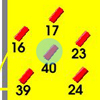Throw a 100

Age 7 to 11 Challenge Level:

Can you score 100 by throwing rings on this board? Is there more than way to do it?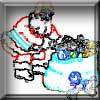Clever Santa

Age 7 to 11 Challenge Level:

All the girls would like a puzzle each for Christmas and all the boys would like a book each. Solve the riddle to find out how many puzzles and books Santa left.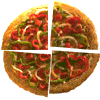Fair Feast

Age 5 to 11 Challenge Level:

Here is a picnic that Petros and Michael are going to share equally. Can you tell us what each of them will have?Tables Teaser

Age 5 to 7 Challenge Level:

How will you work out which numbers have been used to create this multiplication square?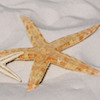Starfish Spotting

Age 5 to 7 Challenge Level:

How many starfish could there be on the beach, and how many children, if I can see 28 arms?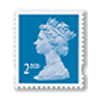Penta Post

Age 7 to 11 Challenge Level:

Here are the prices for 1st and 2nd class mail within the UK. You have an unlimited number of each of these stamps. Which stamps would you need to post a parcel weighing 825g?Napier's Bones

Age 7 to 11 Challenge Level:

The Scot, John Napier, invented these strips about 400 years ago to help calculate multiplication and division. Can you work out how to use Napier's bones to find the answer to these multiplications?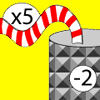Function Machines

Age 7 to 11 Challenge Level:

If the numbers 5, 7 and 4 go into this function machine, what numbers will come out?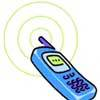Mobile Numbers

Age 5 to 11 Challenge Level:

In this investigation, you are challenged to make mobile phone numbers which are easy to remember. What happens if you make a sequence adding 2 each time?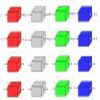Machines

Age 7 to 11 Challenge Level:

What is happening at each box in these machines?Exploring Wild & Wonderful Number Patterns

Age 7 to 11 Challenge Level:

EWWNP means Exploring Wild and Wonderful Number Patterns Created by Yourself! Investigate what happens if we create number patterns using some simple rules.The Deca Tree

Age 7 to 11 Challenge Level:

Find out what a Deca Tree is and then work out how many leaves there will be after the woodcutter has cut off a trunk, a branch, a twig and a leaf.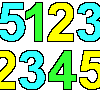Sept03 Sept03 Sept03

Age 7 to 11 Challenge Level:

This number has 903 digits. What is the sum of all 903 digits?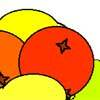Oranges and Lemons

Age 7 to 11 Challenge Level:

On the table there is a pile of oranges and lemons that weighs exactly one kilogram. Using the information, can you work out how many lemons there are?Clock Face

Age 7 to 11 Challenge Level:

Where can you draw a line on a clock face so that the numbers on both sides have the same total?Book Codes

Age 7 to 11 Challenge Level:

Look on the back of any modern book and you will find an ISBN code. Take this code and calculate this sum in the way shown. Can you see what the answers always have in common?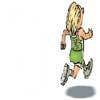Rocco's Race

Age 7 to 11 Short Challenge Level:

Rocco ran in a 200 m race for his class. Use the information to find out how many runners there were in the race and what Rocco's finishing position was.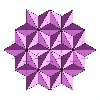Next Number

Age 7 to 11 Short Challenge Level:

Find the next number in this pattern: 3, 7, 19, 55 ...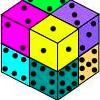Six Numbered Cubes

Age 7 to 11 Challenge Level:

This task combines spatial awareness with addition and multiplication.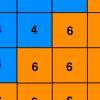Six Ten Total

Age 7 to 11 Challenge Level:

This challenge combines addition, multiplication, perseverance and even proof.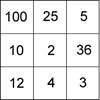Four Go for Two

Age 7 to 11 Challenge Level:

Four Go game for an adult and child. Will you be the first to have four numbers in a row on the number line?Current Playing with Number Upper Primary Teacher

Age 7 to 11 Challenge Level:

Resources to support understanding of multiplication and division through playing with number.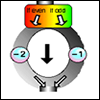The Number Crunching Machine

Age 7 to 11 Challenge Level:

Put a number at the top of the machine and collect a number at the bottom. What do you get? Which numbers get back to themselves?Jumping

Age 7 to 11 Challenge Level:

After training hard, these two children have improved their results. Can you work out the length or height of their first jumps?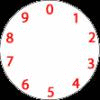Dividing a Cake

Age 7 to 11 Challenge Level:

Annie cut this numbered cake into 3 pieces with 3 cuts so that the numbers on each piece added to the same total. Where were the cuts and what fraction of the whole cake was each piece?Being Resilient - Primary Number

Age 5 to 11 Challenge Level:

Number problems at primary level that may require resilience.The 24 Game

Age 7 to 11 Challenge Level:

There are over sixty different ways of making 24 by adding, subtracting, multiplying and dividing all four numbers 4, 6, 6 and 8 (using each number only once). How many can you find?It Was 2010!

Age 5 to 11 Challenge Level:

If the answer's 2010, what could the question be?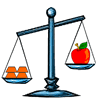What's My Weight?

Age 7 to 11 Short Challenge Level:

There are four equal weights on one side of the scale and an apple on the other side. What can you say that is true about the apple and the weights from the picture?Calendar Calculations

Age 7 to 11 Challenge Level:

Try adding together the dates of all the days in one week. Now multiply the first date by 7 and add 21. Can you explain what happens?Special 24

Age 7 to 11 Challenge Level:

Find another number that is one short of a square number and when you double it and add 1, the result is also a square number.Being Resourceful - Primary Number

Age 5 to 11 Challenge Level:

Number problems at primary level that require careful consideration.Amy's Dominoes

Age 7 to 11 Challenge Level:

Amy has a box containing domino pieces but she does not think it is a complete set. She has 24 dominoes in her box and there are 125 spots on them altogether. Which of her domino pieces are missing?